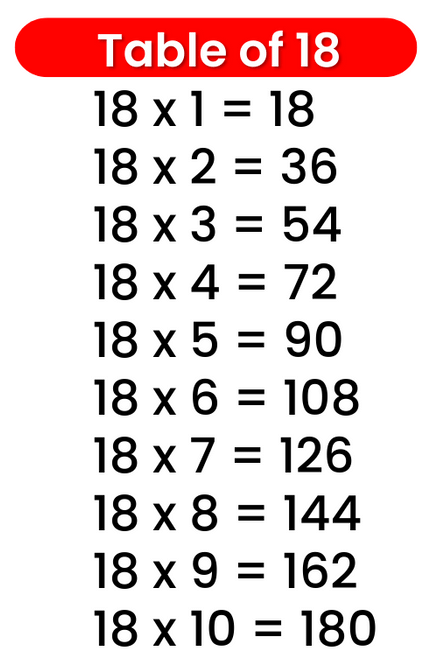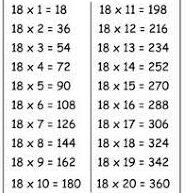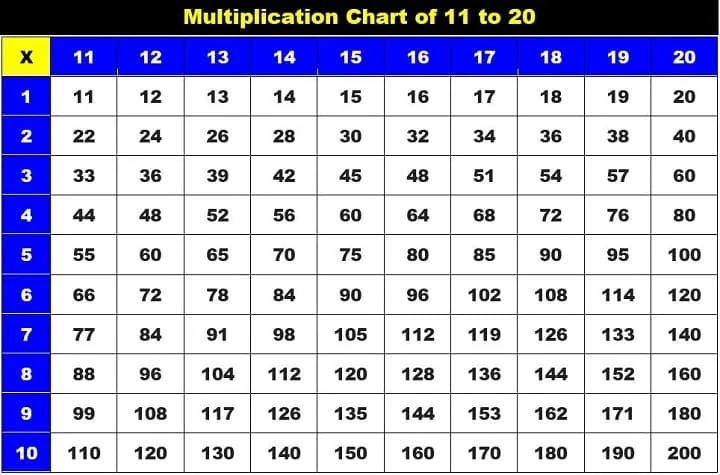# Times Table of 18, 18 ka table

## Times Table of 18, 18 ka table

Maths Multiplication Table of 18, Times Table of 18, 18 ka table, 18 ki Table Chart

18 ×‌ 1 = 18
18 ×‌ 2 = 36
18 ×‌ 3 = 54
18 ×‌ 4 = 72
18 ×‌ 5 = 90
18 ×‌ 6 = 108
18 ×‌ 7 = 126
18 ×‌ 8 = 144
18 ×‌ 9 = 162
18 ×‌ 10 = 180

## 18 ka table### Multiplication Tables of twelve upto 20### Times Table of 18 chart